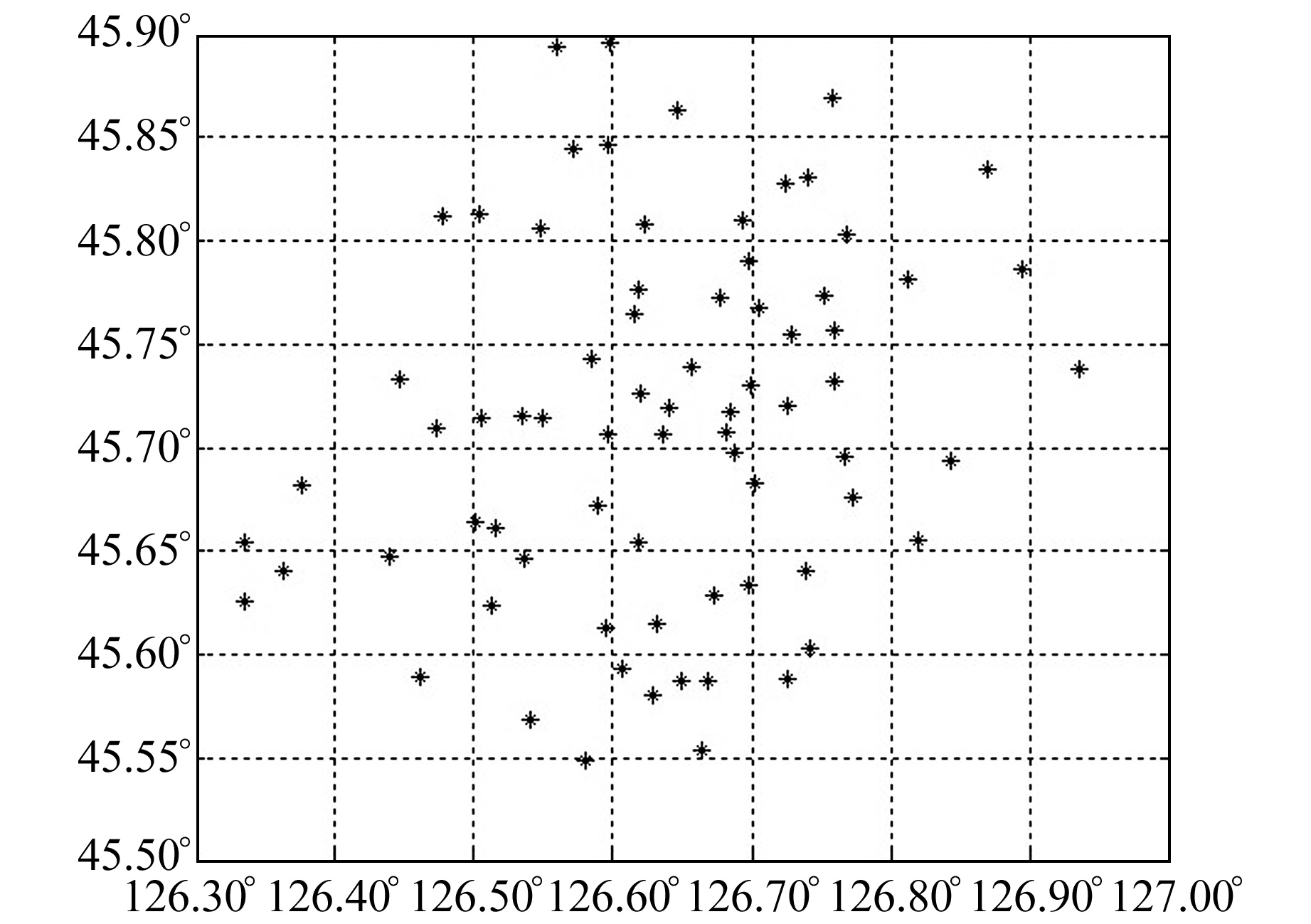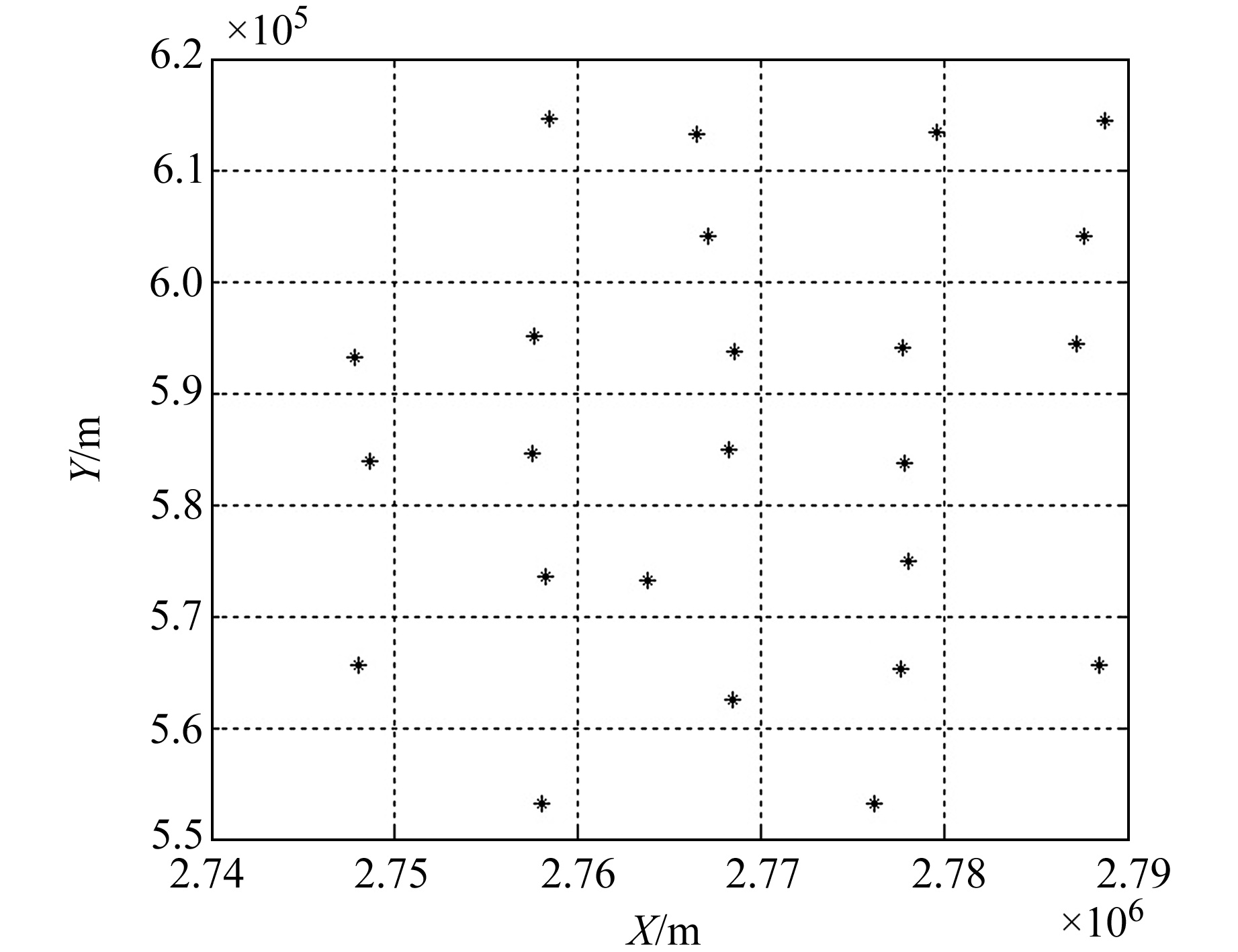﻿ 基于最小二乘支持向量机的组合模型在区域似大地水准面拟合中的应用文章快速检索 高级检索
 大地测量与地球动力学2022, Vol. 42Issue (9): 971-974  DOI: 10.14075/j.jgg.2022.09.016### 引用本文LI Mingfei, WU Junchao, ZHANG Yichi. Application of Combined Model Based on LSSVM in Regional Quasi-Geoid Fitting[J]. Journal of Geodesy and Geodynamics, 2022, 42(9): 971-974.### About the first author

LI Mingfei, engineer, majors in land development and consolidation, E-mail: 1045652087@qq.com.

### 文章历史

1. 陕西省土地工程建设集团有限责任公司，西安市光泰路7号，710075;
2. 华南师范大学地理科学学院，广州市中山大道西55号，510631;
3. 广东省智慧国土工程技术研究中心，广州市中山大道西55号，510631

LSSVM是一种常用的区域似大地水准面拟合方法，其以支持向量机为基础改进而来，在高程转换过程中能够取得较好的效果。但单一的LSSVM会存在模型误差，精度有限，且会造成拟合残差序列信息浪费。基于此，本文以LSSVM为基础，提出LSSVM-Shepard组合区域似大地水准面拟合方法，充分利用拟合的残差序列信息，减少模型误差影响，提高高程转换精度。

1 原理与方法 1.1 LSSVM原理

LSSVM对支持向量机优化方程中的损失函数进行改进，采用误差的二次项之和代替$C \sum\limits_{i=1}^n\left(\varepsilon_i+\varepsilon_i^*\right)$，并将约束条件改为等式约束[3-4]，从而简化模型以提高运算效率。但LSSVM在计算过程中仍会保留核函数环节，与支持向量机一样，其能够有效处理非线性问题。

 \begin{aligned} &\min _{\boldsymbol{\omega}, b, e} J(\boldsymbol{\omega}, e)=\frac{1}{2} \boldsymbol{\omega}^{\mathrm{T}} \boldsymbol{\omega}+\frac{1}{2} \gamma \sum\limits_{i=1}^n e_i^2 \\ &\text { s. t } \quad y_i=\boldsymbol{\omega}^{\mathrm{T}} \boldsymbol{\varphi}\left(x_i\right)+b+e_i, \\ & \ \ \ \ \ \ \ \ \ \ \ \ \ i=1, 2, 3, \cdots, n \end{aligned} (1)

 $\begin{gathered} L(\boldsymbol{\omega}, b, e, \alpha)=J(\boldsymbol{\omega}, e)- \\ \sum\limits_{i=1}^n \alpha_i\left\{\boldsymbol{\omega}^{\mathrm{T}} \boldsymbol{\varphi}\left(x_i\right)+b+e_i-y_i\right\} \end{gathered}$ (2)

 $\left\{\begin{array}{l} \boldsymbol{\omega}=\sum\limits_{i=1}^n \alpha_i \boldsymbol{\varphi}\left(x_i\right), \sum\limits_{i=1}^n \alpha_i=0 \\ \alpha_i=\gamma_{e_i}, \boldsymbol{\omega}^{\mathrm{T}} \boldsymbol{\varphi}\left(x_i\right)+b+e_i-y_i=0 \end{array}\right.$ (3)

 $\left[\begin{array}{ccccc} 0 & 1 & 1 & \cdots & 1 \\ 1 & K\left(x_1, x_1\right)+1 / \gamma & K\left(x_1, x_2\right) & \cdots & K\left(x_1, x_n\right) \\ 1 & K\left(x_2, x_1\right) & K\left(x_2, x_2\right)+1 / \gamma & \cdots & K\left(x_2, x_n\right) \\ \vdots & \vdots & \vdots & \vdots & \\ 1 & K\left(x_n, x_1\right) & K\left(x_n, x_2\right) & \cdots & K\left(x_n, x_n\right)+1 / \gamma \end{array}\right]\left[\begin{array}{c} b \\ \alpha_1 \\ \alpha_2 \\ \vdots \\ \alpha_n \end{array}\right]=\left[\begin{array}{c} 0 \\ y_1 \\ y_2 \\ \vdots \\ y_n \end{array}\right]$ (4)

 $y(x)=\sum\limits_{i=1}^n \alpha_i K\left(x_i, x\right)+b$ (5)
1.2 Shepard插值模型

Shepard插值模型是针对大量离散点提出的局部逼近模型，是一种改进的加权平均法。该模型应用于高程转换的主要原理为：对于给定的数据集(xi, yi, ξi)，i=1, 2，…，n，(xi, yi)为平面位置坐标，ξi为对应的高程异常，则未知点高程异常可通过下式进行求解：

 $\xi(x, y)=\left\{\begin{array}{l} \frac{\sum\limits_{i=1}^n \xi_i\left(\rho\left(r_i\right)\right)^u}{\sum\limits_{i=1}^n\left(\rho\left(r_i\right)\right)^u}, r_i \neq 0 \\ \xi_i, r_i=0 \end{array}\right.$ (6)

 $\rho(r)=\left\{\begin{array}{l} \frac{1}{r}, 0R \end{array}\right.$ (7)

1.3 LSSVM-Shepard组合模型

 $\xi=\xi_{\text {长 }}+\xi_{\text {中 }}+\xi_{\text {短 }}$ (8)

LSSVM将最小二乘思想引入到支持向量机，趋势面变化相对平缓，同时保持支持向量机良好的非线性问题处理能力，可提高计算效率。与二次曲面拟合“移去-恢复”模型的中长波项相比，LSSVM能够更好地处理中长波项中存在的非线性问题。Shepard插值模型可以充分利用周围已知点信息，能够有效处理局部变化信息。本文将两个单一模型进行结合，采用LSSVM拟合高程异常的中长波项，利用Shepard插值模型拟合包含模型误差的短波项，综合两个单一模型的优点，同时充分利用单一模型的拟合残差信息。其主要步骤如下：

1) 利用LSSVM对高程异常的中长波项进行拟合，确定模型系数，并计算包含LSSVM模型误差和短波项的残差序列。

2) 利用Shepard插值模型对残差序列进行训练，使用交叉验证方法确定模型系数。

3) 利用组合模型计算区域内任意一点的高程异常值。

2 算例分析 2.1 项目概况图 1 平原地区GPS点位分布 Fig. 1 Distribution of GPS points in plain area图 2 高原山区GPS点位分布 Fig. 2 Distribution of GPS points in plateau mountainous area
2.2 参数选取

LSSVM模型采用径向基函数作为核函数，其公式为：K(x, xi)=exp(－‖xxi2/2σ2)。该模型含有2个参数，分别为惩罚系数γ和核函数参数σ，模型参数以双层方格网法进行寻优。同时为减少取样随机性所产生的训练偏差，采用交叉验证法进行参数精度评定，将原始数据分为n等份，通过n次循环，每次以其中一份作为测试数据，其余n－1份作为训练数据，得到模型并对测试数据进行预测。统计测试结果，最终获得n次统计结果的平均值，并以此作为参数的评价因子。Shepard插值模型参数为拟合度u和影响半径R，为防止模型陷入局部最优，文中将uR范围分别设置为[1, 50]和[0.1, 20]，搜索步长分别设置为1和0.02，同时通过循环操作使寻优过程遍历每一个节点。

2.3 实验对比分析表 1 平原地区似大地水准面拟合结果统计 Tab. 1 Statistics of fitting results of quasi-geoid in plain area表 2 高原山区似大地水准面拟合结果统计 Tab. 2 Statistics of fitting results of quasi-geoid in plateau mountainous area

3 结语

1) 无论是在平原地区还是高原山区，LSSVM-Shepard模型的拟合效果均优于二次曲面法、LSSVM、Shepard插值等3种单一模型，是一种可行的似大地水准面拟合方法。

2) 二次曲面-Shepard模型在平原实验区进行高程转换时，能够取得较好的拟合效果，但在高原山区实验中，转换度低于Shepard插值模型，适用性受限。LSSVM-Shepard模型以LSSVM代替二次曲面模型，能够有效处理转换过程中的非线性问题，在两个实验中均能取得较好的高程转换效果，具有较强的适用性。

  甘勇, 刘新新, 郑远攀. CPSO优化FLS-SVM应用于山区地形的GPS高程拟合研究[J]. 测绘通报, 2014(4): 5-9 (Gan Yong, Liu Xinxin, Zheng Yuanpan. A Study of GPS Height Fitting in Mountainous Terrain by CPSO Optimizing FLS-SVM[J]. Bulletin of Surveying and Mapping, 2014(4): 5-9) (0)  周理含. 最小二乘支持向量机在GPS高程转换中的应用[J]. 工程地球物理学报, 2010, 7(2): 243-247 (Zhou Lihan. Least Square Support Vector Machine in GPS Height Conversion[J]. Chinese Journal of Engineering Geophysics, 2010, 7(2): 243-247 DOI:10.3969/j.issn.1672-7940.2010.02.021) (0)  李明飞, 吴军超. 基于混合核函数的LSSVM在GNSS高程拟合中的应用[J]. 工程勘察, 2019, 47(12): 64-68 (Li Mingfei, Wu Junchao. Application of LSSVM Based on Hybrid Kernel Functions in GNSS Height Fitting[J]. Geotechnical Investigation and Surveying, 2019, 47(12): 64-68) (0)  Suykens J A K, Vandewalle J. Least Squares Support Vector Machine Classifiers[J]. Neural Processing Letters, 1999, 9(3): 293-300 DOI:10.1023/A:1018628609742 (0)  马文涛. 基于灰色最小二乘支持向量机的边坡位移预测[J]. 岩土力学, 2010, 31(5): 1670-1674 (Ma Wentao. Forecasting Slope Displacements Based on Grey Least Square Support Vector Machines[J]. Rock and Soil Mechanics, 2010, 31(5): 1670-1674 DOI:10.3969/j.issn.1000-7598.2010.05.055) (0)  孙凯, 王颖龙. 支持向量机中Mercer核函数的构造研究[J]. 兵工自动化, 2008, 27(11): 40-42 (Sun Kai, Wang Yinglong. Research on Construction of Mercer Kernel-Function in Support Vector Machine[J]. Ordnance Industry Automation, 2008, 27(11): 40-42 DOI:10.3969/j.issn.1006-1576.2008.11.015) (0)  唐杰明, 刘俊勇, 杨可, 等. 基于灰色模型和最小二乘支持向量机的电力短期负荷组合预测[J]. 电网技术, 2009, 33(3): 63-68 (Tang Jieming, Liu Junyong, Yang Ke, et al. Short-Term Load Combination Forecasting by Grey Model and Least Square Support Vector Machine[J]. Power System Technology, 2009, 33(3): 63-68) (0)  钟波, 罗志才. GPS水准综合模型的应用研究[J]. 测绘通报, 2007(6): 5-7 (Zhong Bo, Luo Zhicai. Research on Application of Combined Model for GPS Leveling[J]. Bulletin of Surveying and Mapping, 2007(6): 5-7 DOI:10.3969/j.issn.0494-0911.2007.06.002) (0)  康靖玉. 综合EGM2008、剩余地势模型及地面重力数据的大地水准面精化[D]. 长春: 吉林大学, 2017 (Kang Jingyu. Geoid Refinement Based on EGM2008, Residual Terrain Model and Ground Gravity Data[D]. Changchun: Jilin University, 2017) (0)  朱君俊. 基于重力场模型EGM2008的高程拟合方法研究[D]. 呼和浩特: 内蒙古农业大学, 2012 (Zhu Junjun. Study on Elevation Fitting Method Based on EGM2008 Model[D]. Hohhot: Inner Mongolia Agricultural University, 2012) (0)  黎剑. 区域GPS高程异常拟合及建模方法研究[D]. 昆明: 昆明理工大学, 2013 (Li Jian. Research on Regional GPS Height Abnormal Fitting and Modeling Method[D]. Kunming: Kunming University of Science and Technology, 2013) (0)
Application of Combined Model Based on LSSVM in Regional Quasi-Geoid Fitting
LI Mingfei1WU Junchao2,3     ZHANG Yichi1
1. Shaanxi Provincial Land Engineering Construction Group Co Ltd, 7 Guangtai Road, Xi'an 710075, China;
2. School of Geography, South China Normal University, 55 West-Zhongshan Road, Guangzhou 510631, China;
3. Guangdong Provincial Center for Smart Land Research, 55 West-Zhongshan Road, Guangzhou 510631, China
Abstract: Aiming at the big model error of least squares support vector machine(LSSVM) in regional quasi-geoid fitting, we establish the combination model of LSSVM and Shepard interpolation model for GPS elevation conversion. We use LSSVM to fit the medium-long wave term in abnormal height, and use Shepard interpolation model to generalize and remove the residual of medium-long wave term. Combined with engineering examples in plain area and plateau mountainous area, we use the quadric surface model, the LSSVM, the Shepard interpolation model, the quadratic-Shepard model, and the LSSVM-Shepard model for elevation conversion and accuracy comparison. The results show that the accuracy of new combined model is higher than that of single model. Moreover, the conversion effect of LSSVM-Shepard model in the plain area is basically consistent with the quadratic-Shepard model, and the fitting effect is better than the quadratic-Shepard model in the plateau mountainous area.
Key words: LSSVM; Shepard interpolation model; quasi-geoid; elevation conversion